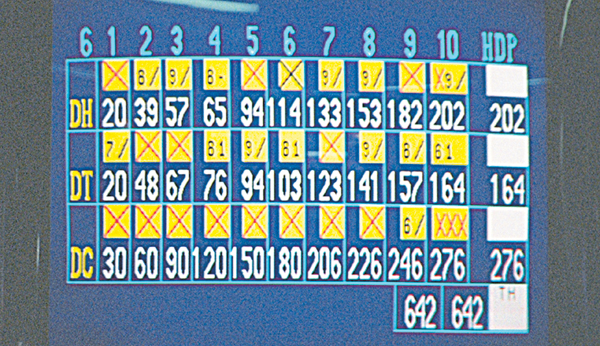# Ten-Pin Bowling – Scoring

In case of knocking down pins, a player scores one point for every knocked-down pin. As there are two chances in a single frame, the combined score of both the chances are the final score of that frame. In case, a player fails to knock down all ten pins even after the two chances of a frame, the frame is called an open frame.

If all the ten pins are knocked down in a single frame, bonuses are awarded to the player based on the number of chances taken by him to knock down all pins. If all ten pins are knocked down by the player in the first chance itself, it is considered as a strike. A strike is specified with a “X” symbol in the score sheet.In case of a player scoring a strike, he gets 10 points for the knocked down pins plus whatever is scored in the next two balls as bonus point. In this way, the points scored in the next two frames of the strike are counted twice. The bowler gets a chance to play two extra balls in case of achieving a strike in the first ball of the 10th frame and one extra ball in case of scoring a spare in the second chance.

For example, if in the first frame the player hits a strike and in the second frame’s first chance he scores 5 and in the second chance of the same frame, he score 3, his total score for the first frame will be 10 + 5 + 3 = 18 and for the second frame, his score will be 5 + 3 = 8 thus making a total score of 18 + 8 = 26.

## Double

In case of two consecutive strikes in two consecutive frames, a player scores a double. In case of a double, the scoring of the player can be explained through an example. Suppose Frame 1 = 10 (Strike) and frame 2 = 10 (Strike), then in frame 3, if the player hits thee pins in the first chance and four pins in the second chance, the total will be calculated as follows.

• Frame 1 Total = 10 + 10 + 3 = 23
• Frame 2 Total = 10 + 3 + 4 = 17
• Frame 3 Total = 3 + 4 = 7
• Total score from 3 frames are 23 + 17 + 7 = 47

## Triple

If a player scores three consecutive strikes in three frames, he scores a Turkey or Triple. In case of a turkey, the total scoring is explained below. Suppose Frame 1 = 10 (Strike), frame 2 = 10(Strike) and frame 3 = 10(Strike) then in frame 4 if the player hits thee pins in the first chance and four pins in the second chance. Then the totals will be −

• Frame1 Total = 10 + 10 + 10 = 30
• Frame2 Total = 10 + 10 + 3 = 23
• Frame3 Total = 10 + 3 + 4 = 17
• Frame4 Total = 3 + 4 = 7
• Total score will be 30 + 23 + 17 + 7 = 77

## Long Consecutive Strikes

The longer consecutive strikes are called by various names like Llama (4 consecutive), pack (6 consecutive) mostly by local languages. Six strikes in a row are referred as wild turkeys, whereas nine strikes in a row are referred as golden turkeys. A game with 12 strikes is rare and is called a perfect game. It is also referred as thanksgiving turkey.

## Clean Game

A clean game is a game in which a player shots at least one strike or spare in each frame. The maximum points that can be scored from a strike is 30. In case of the 10th frame, if the player scores a strike, he/she will be awarded two more chances. If he again scores two strikes, for that frame he will be awarded 30 points which is also called striking out.

In case of a spare, when the player knocks out all pins in the 2nd chance of a frame, the player will be awarded 10 points plus the points scored in the next chance as a bonus. For example, in the first chance of frame, a player hits six pins and in second chance he hits four pins, then he has scored a spare. Now in frame two if the player hits three pins in the first chance and four pins in the second chance, the totals will be −

• Frame 1 total = 6 + 4 + 3 = 13
• Frame 2 total = 3 + 4 = 7
• The total score will be 13 + 7 = 20 for the two frames.

The maximum score that can be scored in a single game is 300 with 12 consecutive strikes which is also called a perfect game. In the bowling score machine, different symbols are shown for different instances. Here “X” stands for a strike, “/” stands for a spare. A – (dash) symbol signifies no pins were knocked down in that frame whereas F indicates a foul.